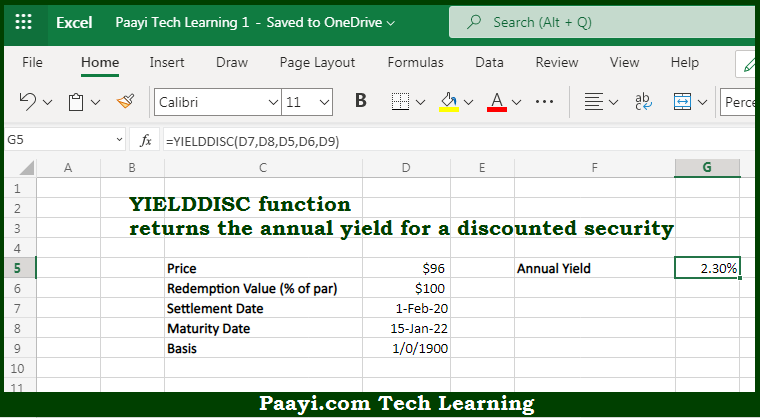# Learn How to Use Microsoft Excel YIELDDISC Function

Written by | 0 Comments | 608 Views

In this article, you will learn how to use the Microsoft Excel YIELDDISC function and its prime function in Microsoft Excel. You will also get to know the Microsoft Excel YIELDDISC function return value and syntax with the help of some examples.

Microsoft Excel YIELDDISC Function

The main purpose of the Microsoft Excel YIELDDISC function is to get the yield for the discounted security. That implies, with the help of the YIELDDISC function you can able to return the annual yield for a discounted security, such as a Treasury bill, that is issued at a discount but that matures at face value. So, with the help of the YIELDDISC function, you can able to get the yield for the discounted security.

Return Value of YIELDDISC Function

The return value will be the yield in the form of a percentage.

Syntax of YIELDDISC Function

=YIELDDISC(sd, md, pr, redemption, [basis])

Where the arguments:

• sd: This is the settlement date of the security.
• md: This is the maturity date of the security.
• pr: This is the price of a security.
• redemption: This is the redemption value per \$100 face value.
• basis: This is the day count basis, and the default is 0 (optional).

How to Use Microsoft Excel YIELDDISC Function?So we know that Microsoft Excel YIELDDISC function you can able to get the yield for the discounted security. That implies, with the help of the YIELDDISC function you can able to return the annual yield for a discounted security, such as a Treasury bill, that is issued at a discount but that matures at face value. So, with the help of the YIELDDISC function, you can able to get the yield for the discounted security.